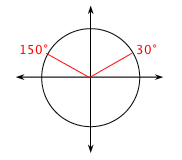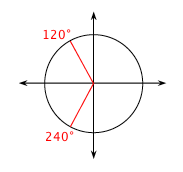### Home > A2C > Chapter 13 > Lesson 13.1.3 > Problem13-46

13-46.

Solve each equation for $0°≤θ<360°$, or if you prefer, $0 ≤ θ < 2π$. You may need your calculator, but remember that it only gives one answer.

1. $\sin(\theta)=0.5$

Use inverse sine on your calculator to find one solution.
sin−1$(0.5) =$ θ

It should tell you the answer is $30°$.

Draw a unit circle to find the other angle that makes sin(θ) $= 0.5$. The sine function is positive in quadrants I and II, so draw a reference angle of $30°$in each quadrant.1. $\cos(\theta)= -0.5$

Follow the steps in part (a).1. $4\tan(\theta)- 4 = 0$

First solve the equation for tan(θ).
tan(θ) $= 1$.

Follow the steps in part (a).

$45°$and $225°$

1. $3\sin^2(\theta)= 1$

First solve the equation for sin(θ).

$\sin(\theta) \ = \pm \sqrt{\frac{1}{3}}$

Follow the steps in part (a). There are $4$ solutions.